Courses

# Test: Communication Systems - 4

## 25 Questions MCQ Test Communication Theory | Test: Communication Systems - 4

Description
This mock test of Test: Communication Systems - 4 for Electronics and Communication Engineering (ECE) helps you for every Electronics and Communication Engineering (ECE) entrance exam. This contains 25 Multiple Choice Questions for Electronics and Communication Engineering (ECE) Test: Communication Systems - 4 (mcq) to study with solutions a complete question bank. The solved questions answers in this Test: Communication Systems - 4 quiz give you a good mix of easy questions and tough questions. Electronics and Communication Engineering (ECE) students definitely take this Test: Communication Systems - 4 exercise for a better result in the exam. You can find other Test: Communication Systems - 4 extra questions, long questions & short questions for Electronics and Communication Engineering (ECE) on EduRev as well by searching above.
QUESTION: 1

### 24 telephone channels, each band limited to 3.4 kHz are to be time division multiplexed using PCM. If sampling frequency is 10 kHz and number of quantization levels is 128, the required bandwidth of PCM is

Solution:

Bandwidth ≈ 24 (ln2 128) 10 kHz = 1.68 MHz.

QUESTION: 2

### Resonant circuits are used in

Solution:

RF amplifiers use resonant loads (L and C in parallel).

QUESTION: 3

### The bandwidth of DSB suppressed carrier modulation system when the modulating frequency varies between 500 Hz and 5 kHz is

Solution:

fm = 5 kHz - 500 Hz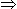4.5 kHz

Bandwidth = 2 fm2 x 4.5 kHz = 9 kHz.

QUESTION: 4

Linear diode detector utilizes

Solution:

Detection (also called demodulation) is due to rectification property of diode.

QUESTION: 5

In the equivalent circuit of a moving coil cone type loudspeaker, the mass of the moving system is represented by

Solution:

Mass has inertia and is analogous to inductance.

QUESTION: 6

Assertion (A): In India we use CCIR-B standard

Reason (R): Video signals are frequency modulated.

Solution:

Video signals are amplitude modulated.

QUESTION: 7

CATV stands for

Solution:

In USA the first cable service was called community antenna television.

QUESTION: 8

A geostationary satellite

Solution:

It orbits the earth in a 24 hour period. Thus it appears to be stationed over one spot of the globe.

QUESTION: 9

Household tape recorders use

Solution:

For reasons of economy only one motor is used.

QUESTION: 10

A 400 W carrier is modulated to a depth of 75 percent. The total power in modulated wave will be

Solution:

Sideband frequencies will be fc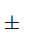fm.

QUESTION: 11

A line has L = 0.8 µH/m and C = 32 pF/m. Z0 =

Solution: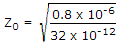.

QUESTION: 12

A line has standing waves with a voltage maximum of 35 mV and minimum of 17.5 mV VSWR is

Solution: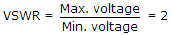.

QUESTION: 13

For a bit rate of 8 kbps, the best possible values of the transmitted frequencies in a coherent binary FSK system are

Solution: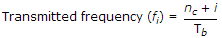where i is integer, and nc is fixed integer.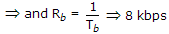fi = Rb(nc + i)(3 + 1) x 832 kHz

(4 + 1)0.840 kHz.

QUESTION: 14

If luminance signal Y = 0.3 R + 0.59 G + 0.11 B, the hue of R - Y colour is

Solution:

R - Y = R - 0.3R - 0.59C - 0.11B = 0.7R - 0.59G - 0.11B.

QUESTION: 15

Trap circuits used in video IF amplifier of colour TV are

Solution:

High Q LC resonant circuit provides high selectivity.

QUESTION: 16

A 1000 kHz carrier is simultaneously modulated with 300 Hz, 800 Hz and 2 kHz audio sine waves. Which of the following frequency is least likely to be present in the output?

Solution:

Frequency present in the sidebands is equal to = fcfm, fc2fm, fc3fm .

QUESTION: 17

An analog signal is band limited to B Hz sampled at Nyquist rate and samples are quantized into 4 levels each with probability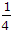. The information rate is

Solution: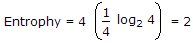.

Signal should be sampled at frequency 2B Hz.

Hence, information rate = 2 (2B) = 4B bits/s.

QUESTION: 18

A 300 Hz sound wave of intensity 20 dB is transmitted by a 1500 kHz radio wave by frequency modulation. It is seen that instantaneous frequency varies between 1500.2 kHz and 1499.8 kHz, 300 times a second. If the intensity of sound wave is 100 dB.

Solution:

Since, the intensity of audio wave has been increased five times, the instantaneous frequency variation is also increased five times.

QUESTION: 19

If the average power of radar transmitters is 2 kW and the peak power of the transmitter is 1000 kW, what will be the duty cycle?

Solution: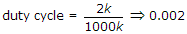.

QUESTION: 20

The HV supply to a TV receiver is 25 kV and the total beam current for the three electron guns is 2000 μA. The power handled by IHVT is

Solution:

25 x 103 x 2000 x 10-6 = 50 W.

QUESTION: 21

Some advantages of optical fibre cables are

1. small diameter
2. Immunity to cross talk and EM interference
3. Laser and LED modulation methods lend themselves ideally to digital operation
Which of the above are correct?
Solution:

Fibre optic cables have all these advantages.

QUESTION: 22

The contrast control in colour TV receiver is done by

Solution:

Luminance amplifier changes the luminance levels and provides contrast control.

QUESTION: 23

The received signal frequency at any time of a super-heterodyne receiver having IF = 456 kHz is 1 MHz. The corresponding image signal is

Solution:

Image signal frequency = Received signal frequency - 2 x Intermediate frequency.

= 1 MHz - 2 x 456kHz1 MHz - 912 kHz88 kHz

which lies in medium bond.

QUESTION: 24

The requirements of carrier frequency are

Solution:

Both should have a precise value and low drift.

QUESTION: 25

If N bits are lumped together to get an N-bit symbol, the possible number of symbols is

Solution:

Each bit can have two levels. Hence 2N.